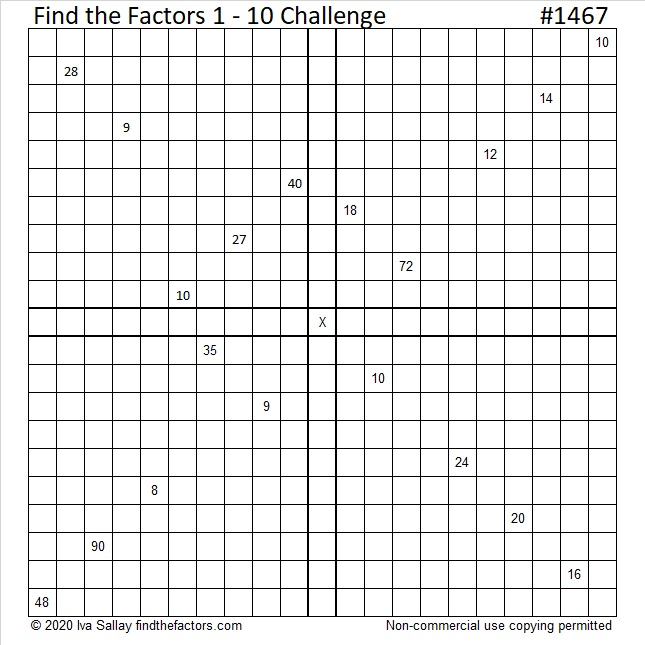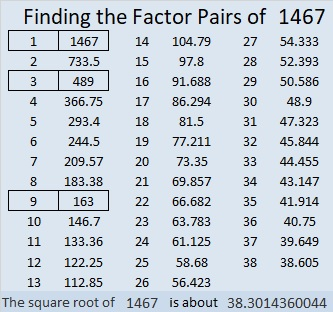# 1467 Challenge Puzzle

### Challenge Puzzle:

Use logic to solve this Challenge Puzzle. The given clues work together to make finding the unique solution a little easier than usual. Have fun with it!Print the puzzles or type the solution in this excel file: 10 Factors 1454-1467

### Factors of 1467:

1467 is divisible by 3 because 1 + 4 = 5 and 5, 6, 7 are three consecutive numbers. Since the middle number, 6, is divisible by 3, we know that 1467 is also divisible by 9.

Of course, you could also add up the digits of 1467 to get 1 + 4 + 6 + 7 = 27, a number divisible by both 3 and 9, to know that 1467 is divisible by both 3 and 9.

• 1467 is a composite number.
• Prime factorization: 1467 = 3 × 3 × 163, which can be written 1467 = 3² × 163
• 1467 has at least one exponent greater than 1 in its prime factorization so √1467 can be simplified. Taking the factor pair from the factor pair table below with the largest square number factor, we get √1467 = (√9)(√163) = 3√163
• The exponents in the prime factorization are 2 and 1. Adding one to each exponent and multiplying we get (2 + 1)(1 + 1) = 3 × 2 = 6. Therefore 1467 has exactly 6 factors.
• The factors of 1467 are outlined with their factor pair partners in the graphic below.